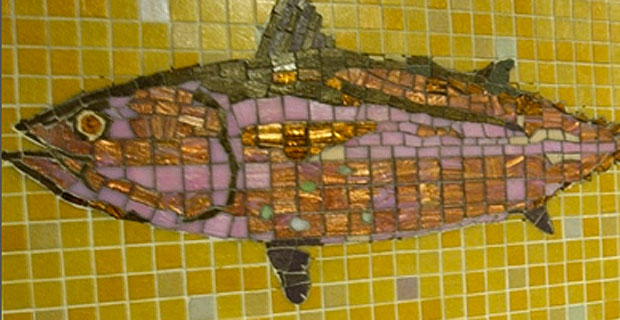# Restaurant Week Menu: The Harrison

Amanda Freitag is at the helm of The Harrison, a local American bistro drawing from seasonal ingredients and also more recently known as a contestant on The Next Iron Chef. The Harrison is offering a summer restaurant menu below:

S U M M E R R E S T A U R A N T W E E K M E N U 2 0 1 0



C H I L L E D C U C U M B E R S O U P

d i l l y o g u r t , s m o k e d p a p r i k a , c a r a w a y c r o u t o n

B I B B L E T T U C E S A L A D

l i m e v i n a i g r e t t e , c r u m b l e d q u e s o b l a n c o

C R I S P Y S K A T E F I N G E R S

n a p a c a b b a g e s l a w & s p i c y c r Ã¨ m e f r a i c h e



B A C O N C R U S T E D B L U E F I S H

p o b l a n o c r e a m & b e e f s t e a k t o m a t o e s

A L L N A T U R A L C H I C K E N B R E A S T

z u c c h i n i , e g g p l a n t , c h i c k p e a s

G R I L L E D F L A N K S T E A K

g r i l l e d c o r n s a l a d & r e d o n i o n j a m



S U M M E R B E R R Y S H O R T C A K E

h o u s e m a d e b i s c u i t s & f r e s h w h i p p e d c r e a m